Courses

# Test: Comparing Quantities- 3

## 20 Questions MCQ Test Mathematics (Maths) Class 8 | Test: Comparing Quantities- 3

Description
This mock test of Test: Comparing Quantities- 3 for Class 8 helps you for every Class 8 entrance exam. This contains 20 Multiple Choice Questions for Class 8 Test: Comparing Quantities- 3 (mcq) to study with solutions a complete question bank. The solved questions answers in this Test: Comparing Quantities- 3 quiz give you a good mix of easy questions and tough questions. Class 8 students definitely take this Test: Comparing Quantities- 3 exercise for a better result in the exam. You can find other Test: Comparing Quantities- 3 extra questions, long questions & short questions for Class 8 on EduRev as well by searching above.
QUESTION: 1

### A sum of money, at compound interest, yields Rs. 200 and Rs. 220 at the end of first and second years respectively. What is the rate percent?

Solution:

We have A=220 and P=200 for the second year
So A=P(1+R100)1
220=200(1+R100)1
11/10-1=R/100
R=10%

QUESTION: 2

Solution:
QUESTION: 3

### The difference in S.I. and C.I. on a certain sum of money in 2 years at 15% p.a. is Rs.144. Find the sum.

Solution:
Let principle be p
T=2years
R=15%
SI= P*R*T/100
=P*2*15/100
=3P/10amount for ci
= P(1+R/100)
=p(1+15/100)
=529/400pCI=529P/400-P
= 529-400/400
= 129/400PCI-SI
=144(given)129p/400-3p/10
=144129p-120p/400
=1449p/100=144p
=144*100/9p
=6400Therefore sum
=6400rs
QUESTION: 4

In what time will a sum of Rs. 800 at 5% p.a. C.I. amount to Rs. 882?

Solution:

A = P(1+R/100)t
882 = 800(1+5/100)t
441/400 = (21/20)t
(21/20)2 = (21/20)t
t = 2 years

QUESTION: 5

What is the C.I. on Rs. 8000 for 1 year at 5% p.a. payable half-yearly?

Solution:

A = P ( 1 + R/100)t. Since its half yearly,
t = 2
= 8000 (1 + 5/200)2
= 8405
CI = A-P = 8405 - 8000 = 405

QUESTION: 6

Raghu borrowed Rs. 25000 at 20% p.a. compounded half-yearly. What amount of money will clear his debt after 112years?

Solution: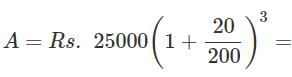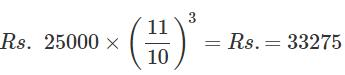QUESTION: 7

In what time will Rs.1000 amount to Rs.1331 at 10% p.a. compounded annually?

Solution: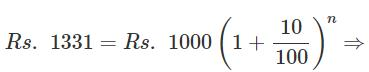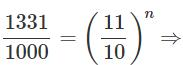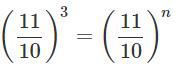⇒ n = 3 years

QUESTION: 8

The present population of a town is 25000. It grows at 4%, 5% and 8% during first year, second year and third year respectively. Find the population after 3 years.

Solution: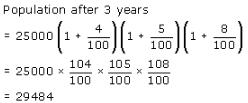QUESTION: 9

The value of an article which was purchased 2 years ago, depreciates at 12% per annum. If its present value is Rs.9680, what is the price at which it was purchased?

Solution: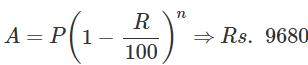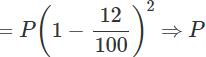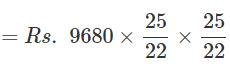= Rs.12500

QUESTION: 10

The cost of a vehicle is Rs.175000. If its value depreciates at the rate of 20% per annum, find the total depreciation after 3 years.

Solution:

Value of the vehicle after 3 years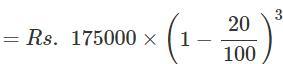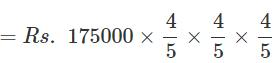= Rs.89600

∴ Total depreciation

= Rs.(175000−89600)

= Rs.85400

QUESTION: 11

The C.I. on a certain sum for 2 years is Rs. 410 and S.I. is Rs. 400. What is the rate of interest per annum?

Solution:

Simple interest for 1 year =400/2=200
CI of 1 year =200
CI for 2nd year=410-200=210
For 2nd year Amount=210 and P=200
A = P (1+R/100)1
210 = 200(1+R/100)
R=5%

QUESTION: 12

The C.I. on a certain sum for 2 years at 10% per annum is Rs. 525. Calculate the S.I. on the same sum for double the time at half the rate percent per annum.

Solution:
QUESTION: 13

The S.I. on a sum of money for two years is Rs. 660, while C.I. is Rs.696.30, the rate of interest being the same in both the cases. Find the rate of interest.

Solution:
QUESTION: 14

A sum of money doubles itself in 3 years at C.I., when the interest is compounded annually. In how many years will it amount to 16 times of itself?

Solution:
QUESTION: 15

The C.I. on a certain sum at 5% for 2 years is Rs. 328. Calculate the S.I. for the sum at the same rate and for the same period.

Solution:

CI = A-P
CI = P(1+R/100)2-P
328 = P[(1+5/100)2-1]
328 = P*41/400
P = 3200
SI = 3200*2*5/100 = 320

QUESTION: 16

Find the amount on Rs.12500 for 2 years compounded annually, the rate of interest being 15% for the first year and 16% for the second year.

Solution: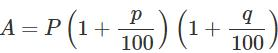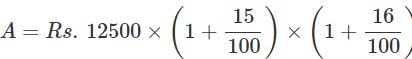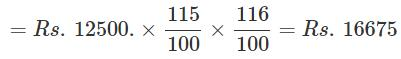QUESTION: 17

Sugar is bought at Rs.16.20 per kg and sold at Rs.17.28  per kg. What is the gain percent?

Solution: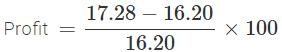QUESTION: 18

A woman bought two packs of toffees, with the same of number of toffees in each pack. She bought the first pack at 25 paise per toffee and the second pack at 3 toffees for 65 paise. She mixed them together and sold at Rs.3.50 a dozen. What is her gain percent?

Solution:

A woman bought 3 toffees at 25 paise each. So she spent 75 paise and 3 for 65 paise, then totally for 6 toffees she spent Rs.1.40 or for a dozen she spent

Rs.2.80 and sold the dozen at Rs.3.50.

∴ Gam

QUESTION: 19

A man bought 542 kg of sugar for Rs. 7560.90and sold it so as to gain 20%. What is the selling price per kilogram of sugar?

Solution:

The cost of sugar per kilogram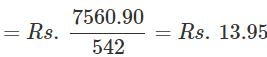S.P. = C.P.(1 + gain%) S.P. at 20% profit = Rs.13.95 × 1.2 = Rs.16.74

QUESTION: 20

A trader marks his goods 30% above the cost price but makes a reduction of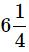on the marked price for ready money. What is his gain percent?

Solution: